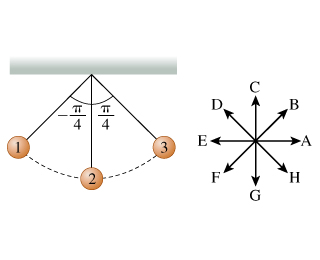# Problem: As the pendulum approaches or recedes from which position(s) is the acceleration vectora→almost parallel to the velocity vectorv→?A. position 2 onlyB. positions 1 and 2C. positions 2 and 3D. positions 1 and 3

###### FREE Expert Solution

The pendulum has both radial acceleration and tangential acceleration. The two are perpendicular. The radial acceleration is directed towards the center of the circle. The tangential acceleration is directed along the tangent of the trajectory. The resultant acceleration lies between the two vectors.###### Problem DetailsAs the pendulum approaches or recedes from which position(s) is the acceleration vector$\stackrel{\mathbf{\to }}{\mathbf{a}}$almost parallel to the velocity vector$\stackrel{\mathbf{\to }}{\mathbf{v}}$?

A. position 2 only

B. positions 1 and 2

C. positions 2 and 3

D. positions 1 and 3